Examples

Chapter 10 Class 12 Vector Algebra
Serial order wise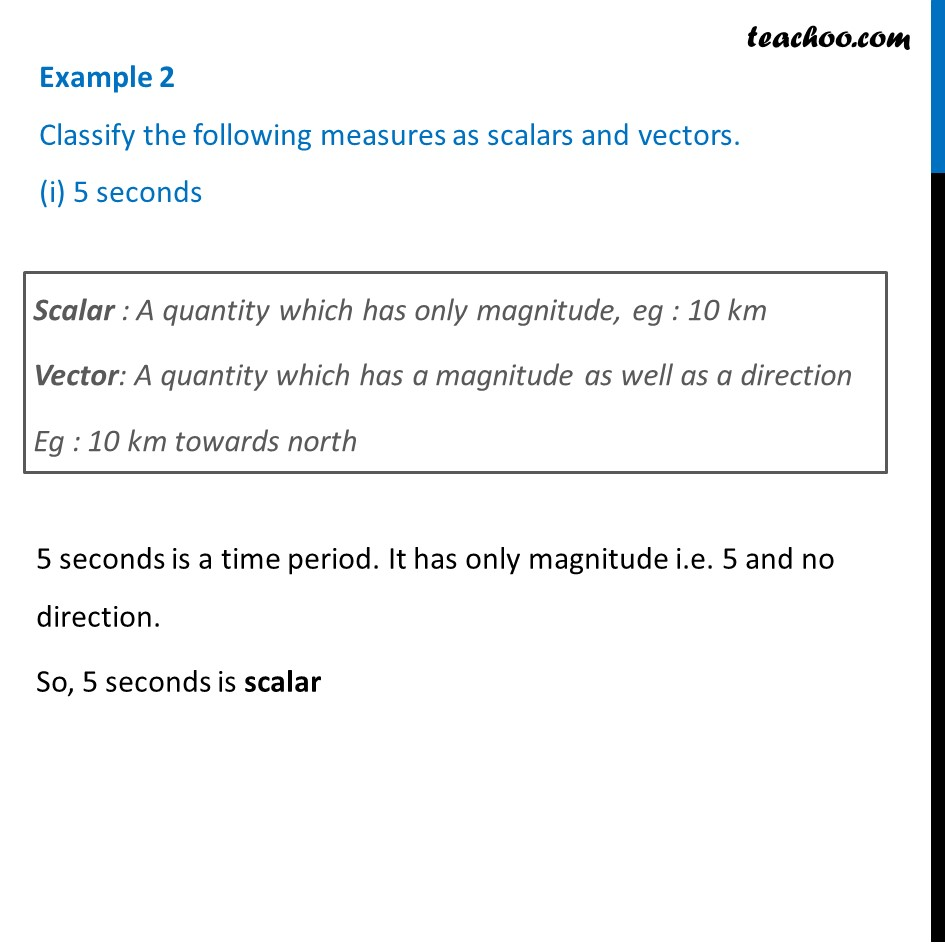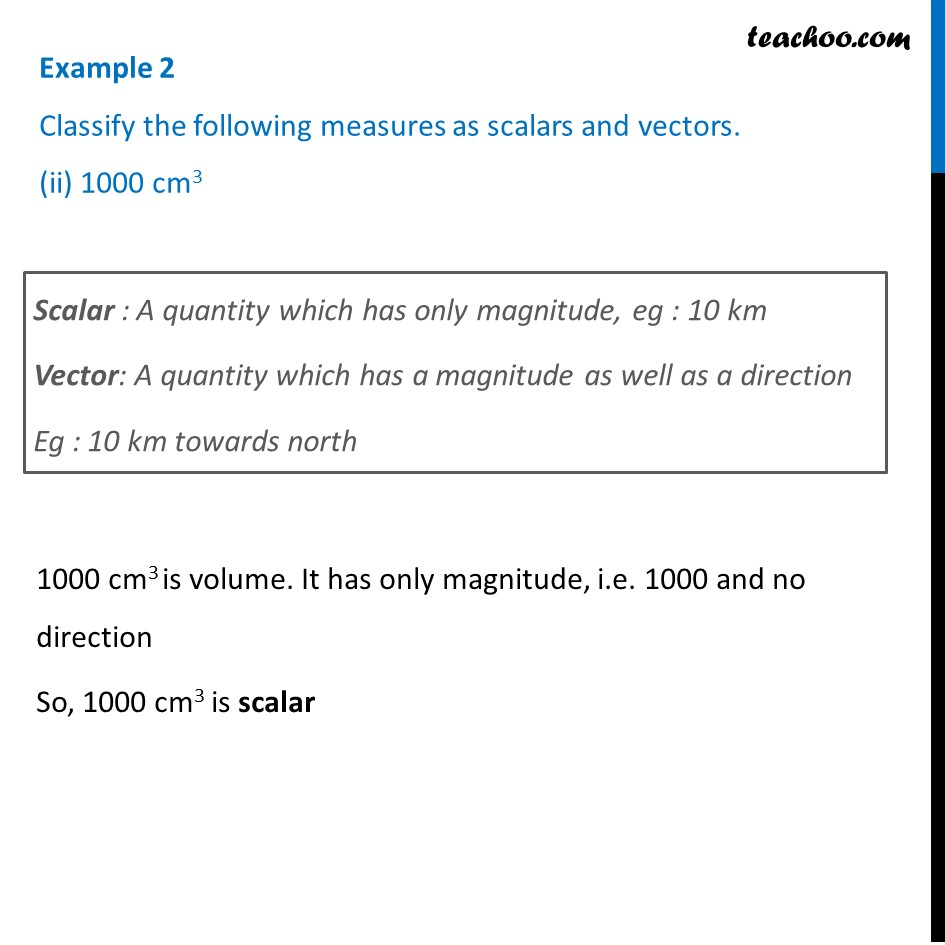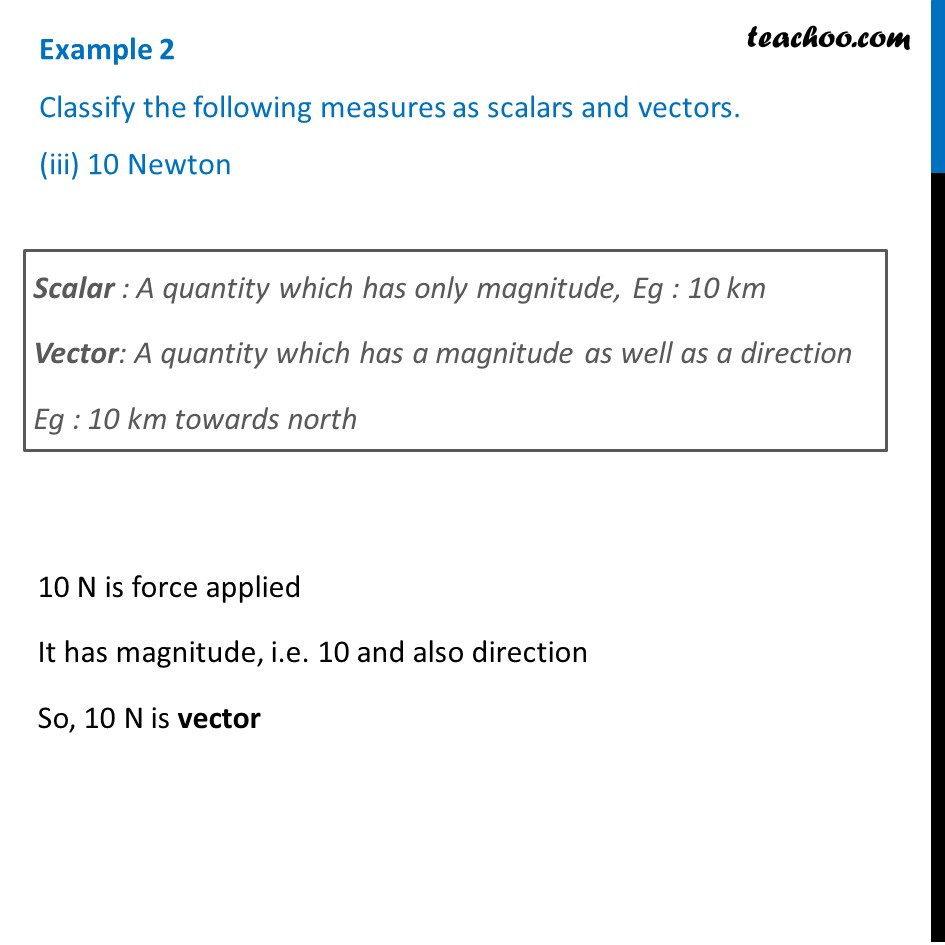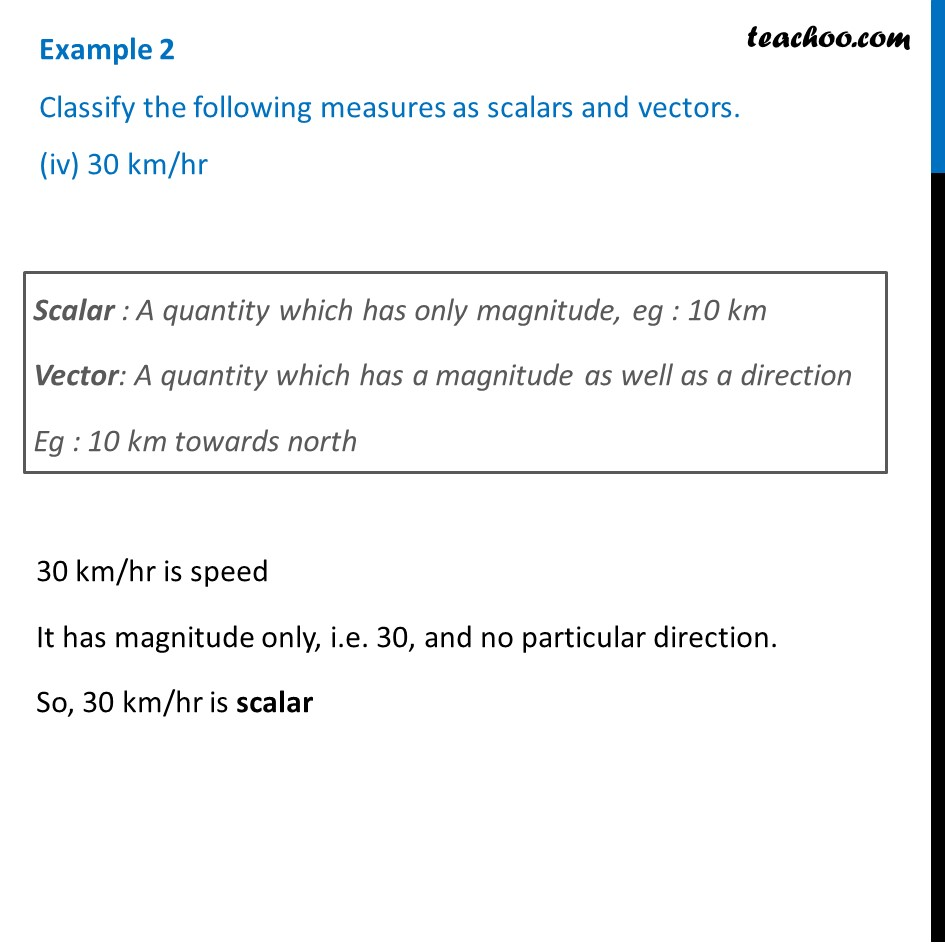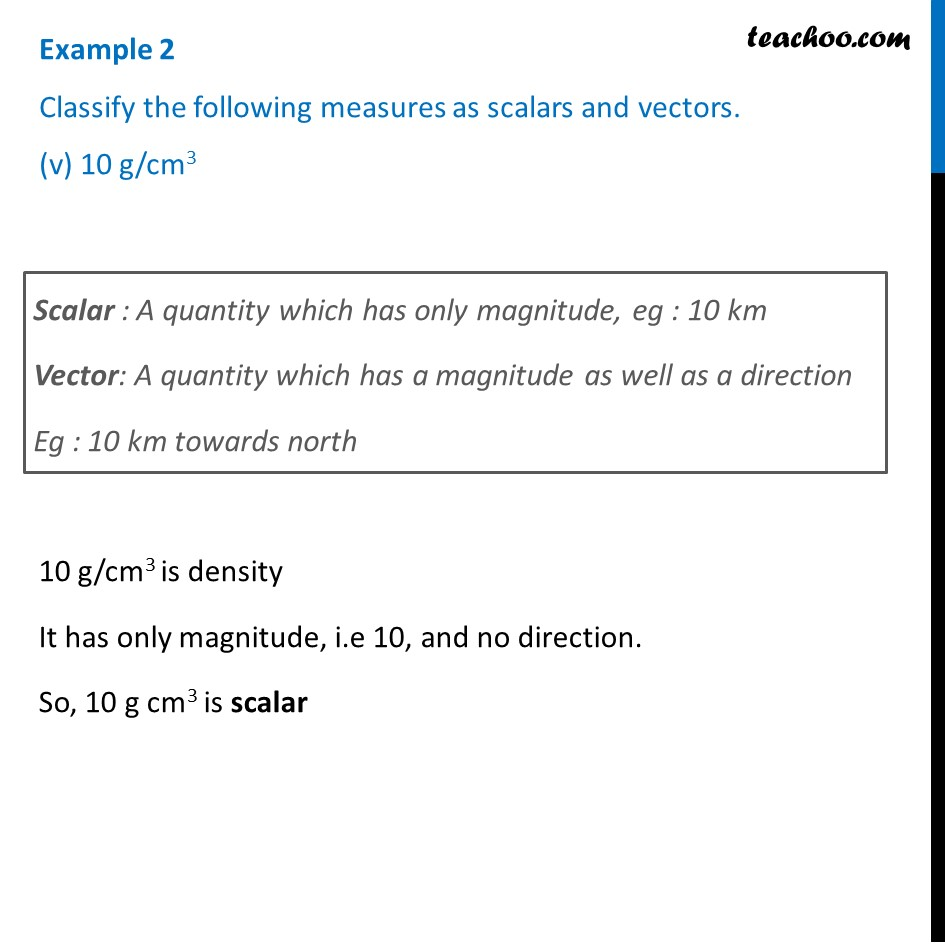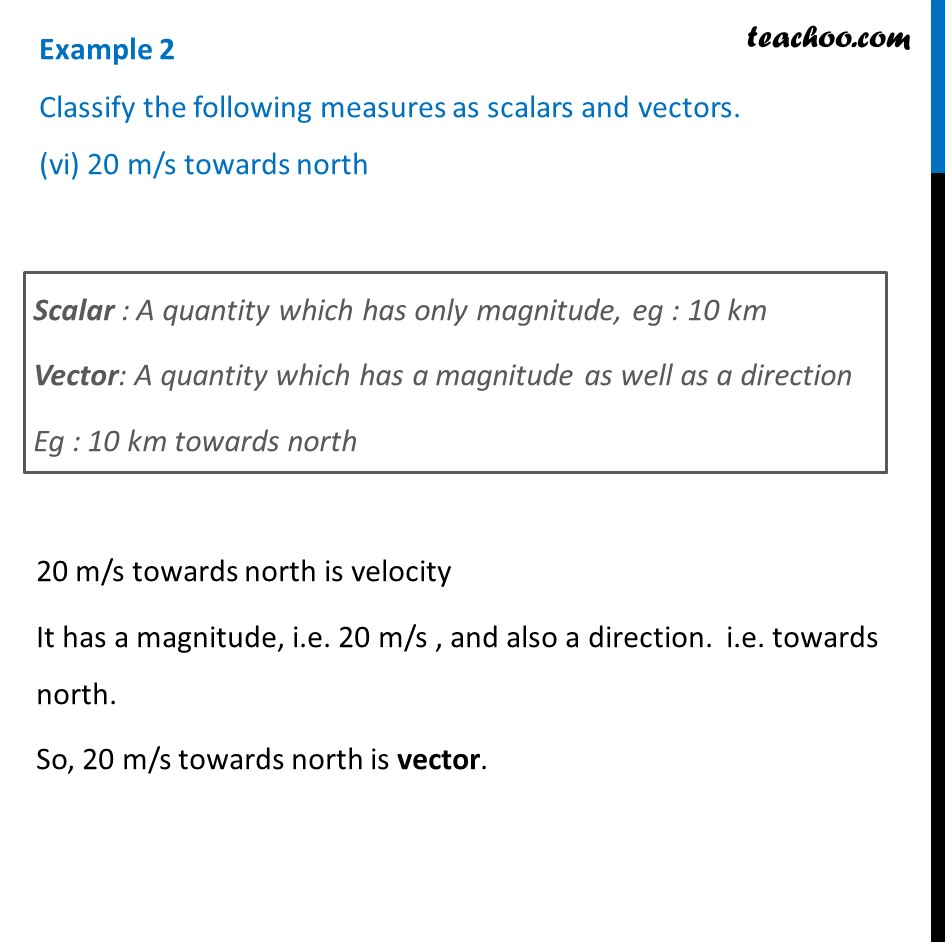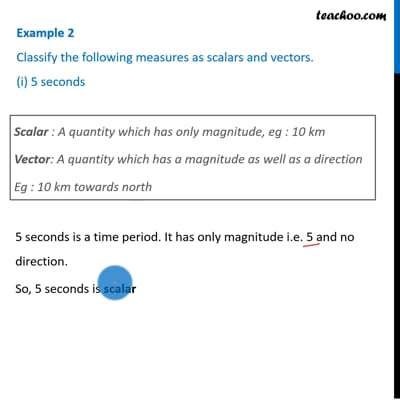This video is only available for Teachoo black users

Introducing your new favourite teacher - Teachoo Black, at only ₹83 per month

### Transcript

Example 2 Classify the following measures as scalars and vectors. (i) 5 secondsScalar : A quantity which has only magnitude, eg : 10 km Vector: A quantity which has a magnitude as well as a direction Eg : 10 km towards north 5 seconds is a time period. It has only magnitude i.e. 5 and no direction. So, 5 seconds is scalar Example 2 Classify the following measures as scalars and vectors. (ii) 1000 cm3 Scalar : A quantity which has only magnitude, eg : 10 km Vector: A quantity which has a magnitude as well as a direction Eg : 10 km towards north 1000 cm3 is volume. It has only magnitude, i.e. 1000 and no direction So, 1000 cm3 is scalar Example 2 Classify the following measures as scalars and vectors. (iii) 10 Newton Scalar : A quantity which has only magnitude, Eg : 10 km Vector: A quantity which has a magnitude as well as a direction Eg : 10 km towards north 10 N is force applied It has magnitude, i.e. 10 and also direction So, 10 N is vectorExample 2 Classify the following measures as scalars and vectors. (iv) 30 km/hr Scalar : A quantity which has only magnitude, eg : 10 km Vector: A quantity which has a magnitude as well as a direction Eg : 10 km towards north 30 km/hr is speed It has magnitude only, i.e. 30, and no particular direction. So, 30 km/hr is scalar Example 2 Classify the following measures as scalars and vectors. (v) 10 g/cm3 Scalar : A quantity which has only magnitude, eg : 10 km Vector: A quantity which has a magnitude as well as a direction Eg : 10 km towards north 10 g/cm3 is density It has only magnitude, i.e 10, and no direction. So, 10 g cm3 is scalar Example 2 Classify the following measures as scalars and vectors. (vi) 20 m/s towards north Scalar : A quantity which has only magnitude, eg : 10 km Vector: A quantity which has a magnitude as well as a direction Eg : 10 km towards north 20 m/s towards north is velocity It has a magnitude, i.e. 20 m/s , and also a direction. i.e. towards north. So, 20 m/s towards north is vector.